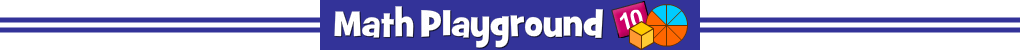Place the fractions on the number line.
Click a fraction then click its place on the number line.
Can you solve all 12 levels?
Number Line Hunt - Learning Connections
Essential Skills
Mental Math - Fractions on a Number Line

Common Core Connection for Grades 3 and 4
Represent a fraction a/b on a number line diagram.
Compare two fractions with different numerators and different denominators.
Recognize that comparisons are valid only when the two fractions refer to the same whole.
More Math Games to Play